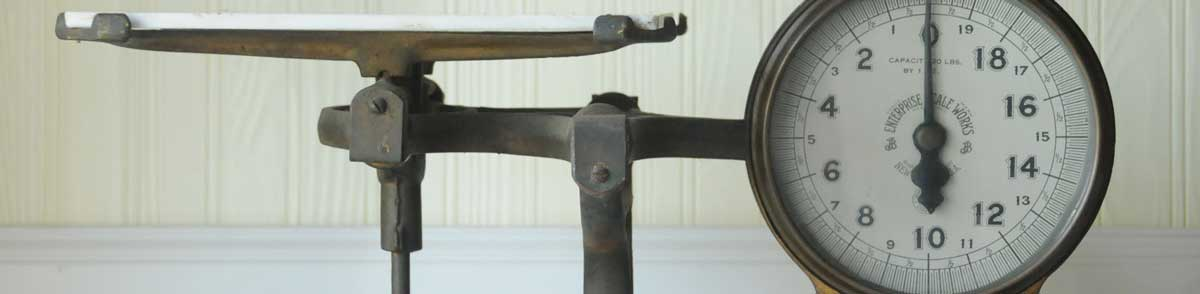Convert Long Tons to Metric Tons (Tonnes)

Enter the weight in long tons below to get the value converted to metric tons.

Results in Metric Tons:1 lt = 1.016047 t

How to Convert Long Tons to Metric TonsTo convert a long ton measurement to a metric ton measurement, multiply the weight by the conversion ratio. One long ton is equal to 1.016047 metric tons, so use this simple formula to convert:

metric tons = long tons × 1.016047

The weight in metric tons is equal to the long tons multiplied by 1.016047.

For example, here's how to convert 5 long tons to metric tons using the formula above.
5 lt = (5 × 1.016047) = 5.080235 t

Long tons and metric tons are both units used to measure weight. Keep reading to learn more about each unit of measure.

Long Tons

One long ton is equal to 2,240 pounds and is mostly used in the United Kingdom as a unit of mass/weight. Long tons are not the same as the short ton, which is used mostly in the United States, or the metric ton which is used throughout the world.

The long ton is an imperial unit of weight. A long ton is sometimes also referred to as an imperial ton or displacement ton. Long tons can be abbreviated as lt, and are also sometimes abbreviated as t. For example, 1 long ton can be written as 1 lt or 1 t.

Metric Tons (Tonnes)

One tonne is equal to 1,000 kilograms or 2,204.6 pounds, and is used throughout the world as a unit of mass/weight. One tonne is also equal to 1 megagram. The metric ton should not be confused with short tons, used primarily in the US, or long tons, used primarily in the UK.

The metric ton is an SI accepted unit for weight for use with the metric system. A metric ton is sometimes also referred to as a tonne. Metric tons can be abbreviated as t, and are also sometimes abbreviated as T, Te, or MT. For example, 1 metric ton can be written as 1 t, 1 T, 1 Te, or 1 MT.

In the US the unit is referred to as the metric ton, while the rest of the world refers to it as the tonne. Most often it's still pronounced as tun, but it is sometimes pronounced tunny.

Long Ton Measurements and Equivalent Metric Ton Conversions

Common long ton values converted to the equivalent metric ton value
Long Tons Metric Tons
1 lt 1.016 t
2 lt 2.0321 t
3 lt 3.0481 t
4 lt 4.0642 t
5 lt 5.0802 t
6 lt 6.0963 t
7 lt 7.1123 t
8 lt 8.1284 t
9 lt 9.1444 t
10 lt 10.16 t
11 lt 11.18 t
12 lt 12.19 t
13 lt 13.21 t
14 lt 14.22 t
15 lt 15.24 t
16 lt 16.26 t
17 lt 17.27 t
18 lt 18.29 t
19 lt 19.3 t
20 lt 20.32 t
21 lt 21.34 t
22 lt 22.35 t
23 lt 23.37 t
24 lt 24.39 t
25 lt 25.4 t
26 lt 26.42 t
27 lt 27.43 t
28 lt 28.45 t
29 lt 29.47 t
30 lt 30.48 t
31 lt 31.5 t
32 lt 32.51 t
33 lt 33.53 t
34 lt 34.55 t
35 lt 35.56 t
36 lt 36.58 t
37 lt 37.59 t
38 lt 38.61 t
39 lt 39.63 t
40 lt 40.64 t

More Long Ton Weight Conversions

US Customary & Imperial Units
Convert to Tons
1 lt is equal to 1.12 tons
Convert to Pounds
1 lt is equal to 2,240 pounds
Convert to Ounces
1 lt is equal to 35,840 ounces
SI Units
Convert to Kilograms
1 lt is equal to 1,016.05 kilograms
Convert to Grams
1 lt is equal to 1,016,046.9 grams
Convert to Milligrams
1 lt is equal to 1,016,046,900 milligrams
Other Units
Convert to Carats
1 lt is equal to 5,080,234.5 carats